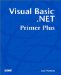# Review Questions

For testing purposes, simply create a new project without placing any controls on the form. Unless stated otherwise , you can place any code statements in the form Load() event for testing purposes.

 1: What is an array scalar and why is it important? A1: An array scalar is used to determine how much storage an array needs. Storage requirements are calculated by taking the number of bytes for each element in the array (that is, the size of the type specifier ) and multiplying it by the number of elements requested . For example, ` Dim MyArray(10) as Double ` needs 88 bytes of storage. To derive this number, take the number of bytes for the type specifier (8 bytes per Double ) and multiply the number of elements (11 because the dimension starts with 0) to arrive at the 88 bytes for the array. 2: Will the following statement produce a syntax error? Why or why not? ` Dim MyArray() as Long ` A2: It will not produce a syntax error. The reason is because the statement defines a dynamic array. At some point in the program before the array is used, however, there needs to be a ReDim statement so that the Windows memory manager can allocate storage for the array. 3: What is the syntax form for sorting an array? A3: The syntax is ` Array.Sort(MyArray) ` This will sort the array named MyArray . 4: What advantage does the Sort() method of the array class have over writing your own sort routine? A4: Any time you write your own sort routine, you must write it in such a way that it compares two data items and then decides whether those two items need to be swapped. Because the comparison is for a specific data type (for example, comparing two Double s), it's impossible to write a generic sort routine that's capable of sorting all data types. Therefore, if you want to sort an array of Integer s and a different array of Double s, you would need to write two sort routines. More code, more opportunity for bugs . Using the built-in Sort() method of the Array class eliminates this problem. Another advantage is that the Sort() method is going to be very efficient. Microsoft is smart enough to know that the Sort() method will likely be used heavily and will optimize the routine as much as possible. Indeed, if you write your own sort routine and time it against the Sort() method, you'll find a noticeable difference in favor of the Sort() method. 5: Although a binary search is an effective search of a data array, what is its major limitation? A5: The disadvantage of a binary search is that the data being searched must be in sorted order. 6: If you have an array that for whatever reason cannot be sorted, and you needed to find a specific value in the array, how would you search the array for that value? A6: One solution is to use the IndexOf() method to perform a sequential search of the array. In cases where the array list is short and not sorted, the IndexOf() method is faster than sorting the array and then using the BinarySearch() method. 7: Suppose a colleague writes some code that uses an array, but you have no idea of the array's dimensions. How could you find out its size? A7: You might use ` Dim i, TheRank, NumElements As Integer Dim MyArray(5, 10) As Integer TheRank = MyArray.Rank If TheRank > 1 Then For i = 1 To TheRank NumElements = UBound(MyArray, i) Next Else NumElements = UBound(MyArray) End If ` In this code, the call to the Rank() method tells you how many dimensions the array has. After all, it could be a multidimensional array. The If test determines which (overloaded) version of UBound() needs to be called. If Rank() returns more than one dimension, a For loop iterates through the ranks to determine the array boundaries. Otherwise, a single call to UBound() is sufficient. You could also use the GetLength() method, too. 8: What is a reference variable and why is it different from other variables ? A8: A reference variable is used to hold the memory address of another variable or data object. That is, the rvalue of a reference variable is designed to hold the memory address of another variable or object. Because of this, the statement ` Dim Aref as Double() ` defines a reference variable that is designed to point to a Double data type. The Aref variable requires 4 bytes of storage because that's how much memory is required to store a memory address. Therefore, even though Aref references a Double data type (which uses 8 bytes of storage), Aref only requires 4 bytes to hold the address of where the Double is stored. 9: In what ways are collections different from arrays and what advantages do collections offer? A9: Collections are useful for storing related information just like arrays, but they have the advantage of being able to store data of differing types. Whereas arrays are limited to storing one type of data (perhaps an array of type Double ), collections can contain dissimilar data items. Further, the Add() and Remove() collection methods make it easier to manage a collection.Visual Basic .NET Primer Plus
ISBN: 0672324857
EAN: 2147483647
Year: 2003
Pages: 238
Authors: Jack Purdum

Similar book on Amazon